# Non Verbal Reasoning - Analogy

### Exercise :: Analogy - Section 2

Each of the following questions consists of two sets of figures. Figures A, B, C and D constitute the Problem Set while figures 1, 2, 3, 4 and 5 constitute the Answer Set. There is a definite relationship between figures A and B. Establish a similar relationship between figures C and D by selecting a suitable figure from the Answer Set that would replace the question mark (?) in fig. (D).

26.

Select a suitable figure from the Answer Figures that would replace the question mark (?).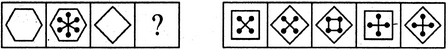(A)     (B)      (C)     (D)                  (1)      (2)      (3)      (4)      (5)

 A. 1 B. 2 C. 3 D. 4 E. 5

Explanation:

As many pins are introduced inside the figure as the number of sides of the figure. These pins start from the centre of the figure and have their heads pointing towards the sides of the figure.

27.

Select a suitable figure from the Answer Figures that would replace the question mark (?).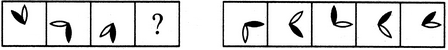(A)     (B)      (C)     (D)                  (1)      (2)      (3)      (4)      (5)

 A. 1 B. 2 C. 3 D. 4 E. 5

Explanation:

The black leaf rotates 135oACW and white leaf rotates 90oACW

28.

Select a suitable figure from the Answer Figures that would replace the question mark (?).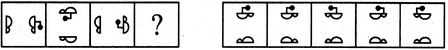(A)     (B)      (C)     (D)                  (1)      (2)      (3)      (4)      (5)

 A. 1 B. 2 C. 3 D. 4 E. 5

Explanation:

The RHS element rotates 90oCW and moves to the upper position. The LHS element rotates 90oCW and moves to the lower position.

29.

Select a suitable figure from the Answer Figures that would replace the question mark (?).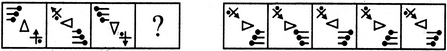(A)     (B)      (C)     (D)                  (1)      (2)      (3)      (4)      (5)

 A. 1 B. 2 C. 3 D. 4 E. 5

Explanation:

The set of three pins gets vertically inverted and moves to the lower-right position; the triangle rotates 90oACW; the third element rotates 45oACW and moves to the upper-left position. The dot in this element moves to the adjacent portion in a CW direction.

30.

Select a suitable figure from the Answer Figures that would replace the question mark (?).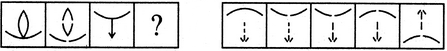(A)     (B)      (C)     (D)                  (1)      (2)      (3)      (4)      (5)

 A. 1 B. 2 C. 3 D. 4 E. 5